## Our Videos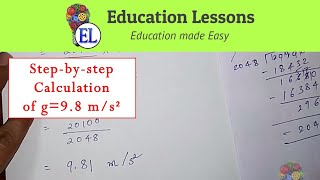Gravitational Acceleration g is 9.8 m/s² - Step by Step Calculation!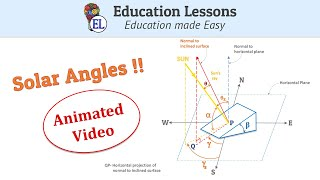Solar Angles [Animated] | Angle of Latitude| Zenith Angle|Azimuth Angle|Tilt &amp; Inclination[English]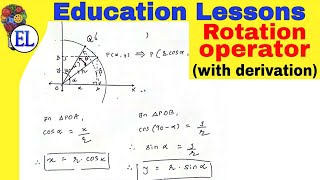Rotation Operator with full Derivation | Vector projection (Vector calculus and linear algebra)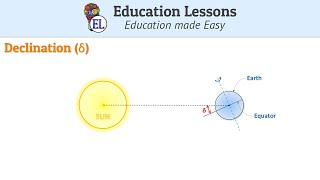Solar Declination angle (δ) | Animated Video [English]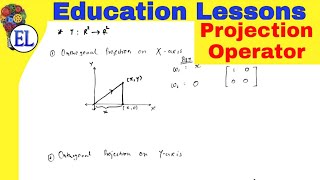Projection Operator | Vector projection (Vector calculus and linear algebra)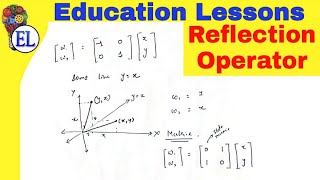Reflection Operator Linear Algebra | Matrix transformation | VCLAAssignment Problem 1 | Hungarian method | Operation Research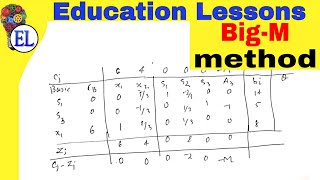Big M Method in Hindi | Linear programming problem | Operation ResearchAssignment Model in Operation Research in Hindi | INTRODUCTION | Assignment Problem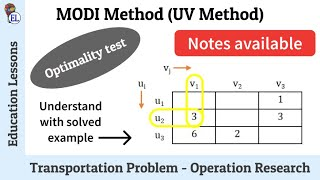Modi Method Transportation problem in Hindi | UV Method Transportation Problem | Operation Research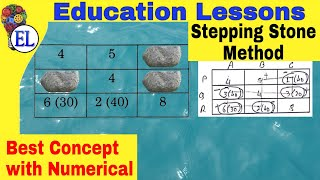Stepping Stone Method transportation problem in Hindi | Transportation Problem | Operation Research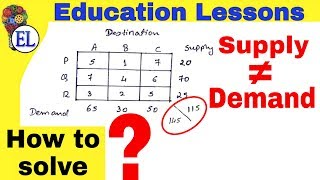Unbalanced Transportation Problem in Hindi | Transportation problem | Operation Research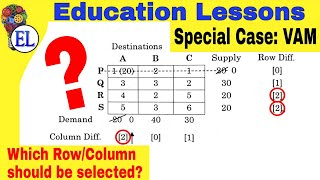Tie in selecting Row and Column | Vogels approximation method in Hindi (VAM)| Transportation Problem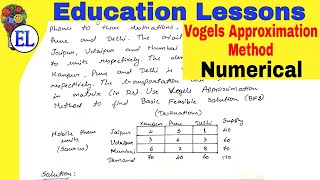Vogels approximation method in Hindi (VAM) | Transportation Problem | Operation Research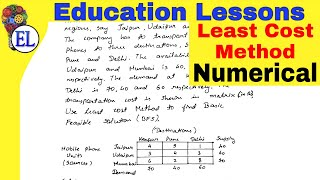Least Cost Method transportation problem in Hindi | Operation Research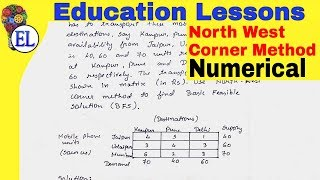North west corner method transportation problem in Hindi | North west corner rule | OR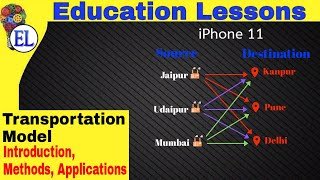Transportation Problem Introduction | Operation research (OR)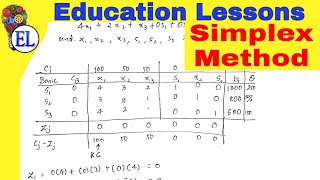Simplex Method in Hindi | Linear programming problem (LPP) by simplex method | Theory+Solved problem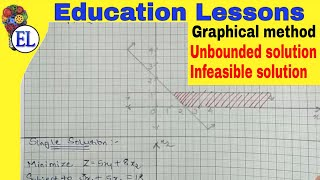LPP | Special cases for Graphical method | Infeasible solution, Unbounded solution | OR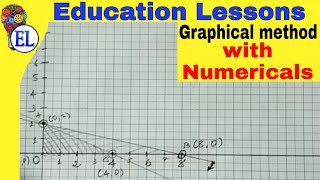Graphical Method in Linear Programming | Maximization and minimization problems | Operation Research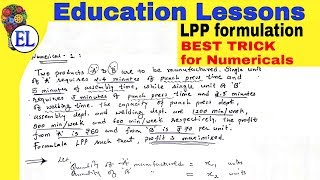LPP Formulation | Theory + Problems | Linear programming problem | Operation Research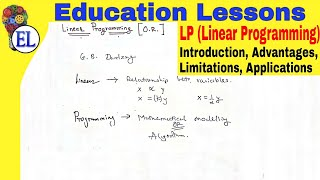LPP | Linear Programming problem in hindi | Introduction, Advantages, Limitations, Applications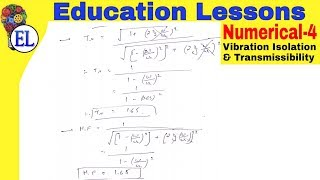Numerical 4 | Vibration Isolation and Transmissibility | Dynamics of machinery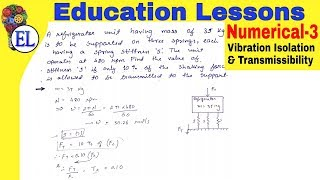Numerical 3 | Vibration Isolation and Transmissibility | Dynamics of machinery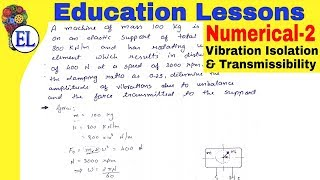Numerical 2 | Vibration Isolation and Transmissibility | Dynamics of machinery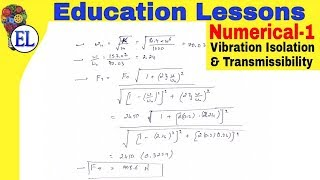Numerical 1 | Vibration Isolation and Transmissibility | Dynamics of machinery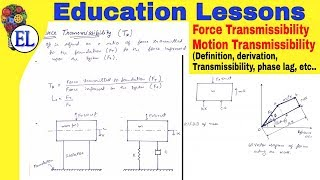Vibration Transmissibility (Force Transmissibility And Motion Transmissibility) Derivation | DOM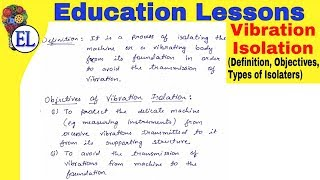Vibration Isolation | Definition, Objectives, Types | Vibration isolation and Transmissibility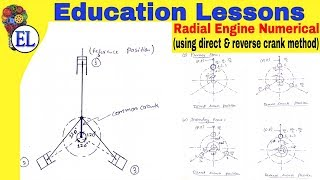Radial Engine Numerical (Using Direct and Reverse Crank method) | Balancing of Reciprocating masses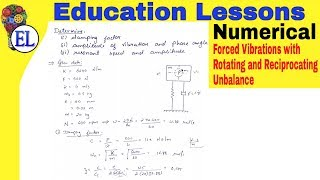Numerical | Forced Vibration with Rotating and Reciprocating Unbalance | Dynamics Of Machinery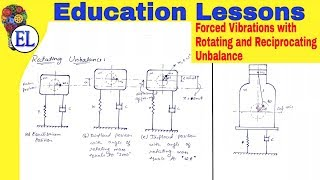Forced Vibration with Rotating and Reciprocating Unbalance | Dynamics of Machinery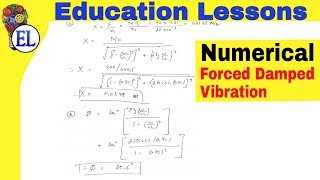Numerical on Forced Damped Vibration with Constant Harmonic Excitation | DOM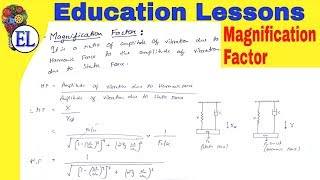Magnification Factor or Dynamic Magnifier | Forced Damped Vibration | DOM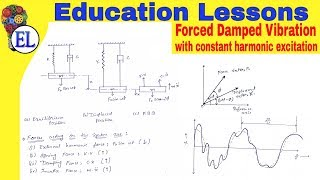Forced Damped Vibration with Constant Harmonic Excitation in Hindi | Dynamics of Machinery (DOM)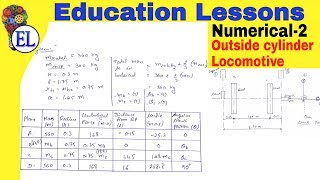Numerical 2 | Balancing of Locomotive Engine | Find Tractive Force, Swaying Couple, etc...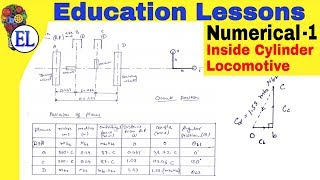Numerical 1 | Balancing of Locomotive Engine | Find Tractive Force, Swaying Couple, etc...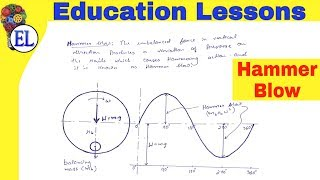Hammer Blow | Effect of Partial Balancing of Locomotives | (DOM)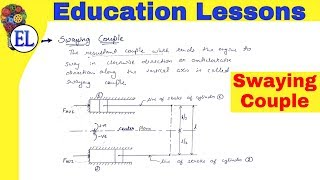Swaying Couple | Effect of Partial Balancing of Locomotives | (DOM)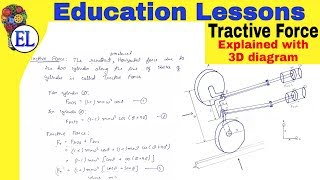Variation of Tractive Force | Effect of Partial Balancing of Locomotives | (DOM) | Tractive Effort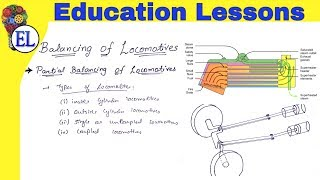Partial balancing of Locomotives | Introduction, Working, Types, Why partial balancing??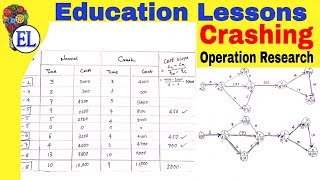Crashing of Project Network in Hindi | Operation Research (OR) | Pert and Cpm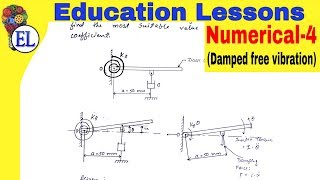Damped Free Vibration Numerical 4 | Dynamics of machinery (DOM) | Hindi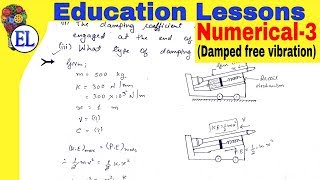Damped Free Vibration Numerical 3 | Dynamics of machinery (DOM) | Hindi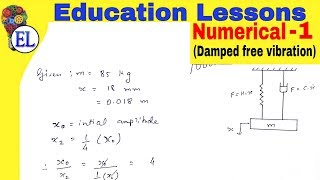Damped Free Vibration Numerical 1 | Dynamics of machinery (DOM) | Hindi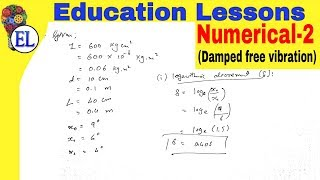Damped Free Vibration Numerical 2 | Dynamics of machinery (DOM) | Hindi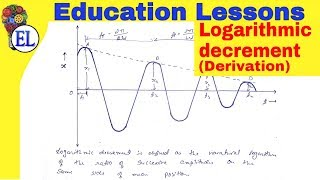Logarithmic Decrement Derivation in Hindi | Damped Free Vibration | Dynamics of machinery (DOM)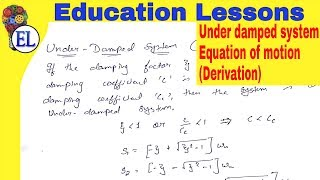Underdamped system | Derivation of equation of motion | Damped free vibrations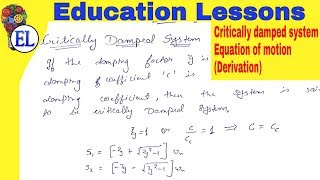Critically damped system | Derivation of equation of motion | Damped free vibrations

## What is Assignment Model?

→ Assignment model is a special application of Linear Programming Problem (LPP), in which the main objective is to assign the work or task to a group of individuals such that;

i) There is only one assignment.

ii) All the assignments should be done in such a way that the overall cost is minimized (or profit is maximized, incase of maximization).

→ In assignment problem, the cost of performing each task by each individual is known.
→ It is desired to find out the best assignments, such that overall cost of assigning the work is minimized.

#### For example:

• Suppose there are 'n' tasks, which are required to be performed using 'n' resources.

• The cost of performing each task by each resource is also known (shown in cells of matrix)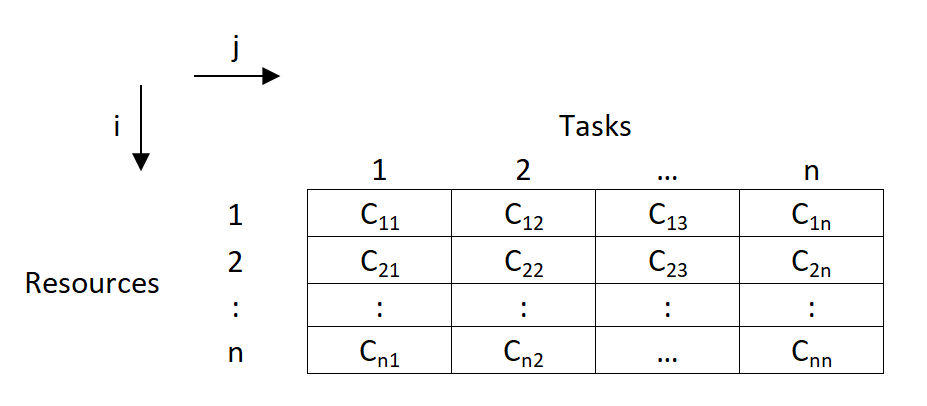• In the above asignment problem, we have to provide assignments such that there is one to one assignments and the overall cost is minimized.

→ Assignment Model is a special application of Linear Programming (LP).

→ The mathematical formulation for Assignment Model is given below:→ Let, $\text {C}_{ij}$ denotes the cost of resources 'i' to the task 'j'; such that

\begin{aligned} x_{ij} &= 1 \ ; \text{if} \ \ i^{th} \text{resource is original to } j^{th} \text{task}\\ x_{ij} &= 0 \ ; \text{if} \ \ i^{th} \text{resource is not original to } j^{th} \text{task} \end{aligned}

→ Now assignment problems are of the Minimization type. So, our objective function is to minimize the overall cost.

$\therefore \text{Minimize Z}= \displaystyle\sum_{j=1}^n \ \sdot \ \displaystyle\sum_{i=1}^n \ C_{ij} \ \sdot \ x_{ij}$

→ Subjected to constraint;

(i) For all $j^{th}$ task, only one $i^{th}$ resource is possible:

$\therefore \displaystyle\sum_{j=1}^n \ x_{ij} = 1 \quad \text{(i=1,2,3, ...,n)}$

(ii) For all $i^{th}$ resource, there is only one $j^{th}$ task possible;

$\therefore \displaystyle\sum_{i=1}^n \ x_{ij} = 1 \quad \text{(j=1,2,3, ...,n)}$

(iii) $x_{ij}$ is '0' or '1'.

## Types of Assignment Problem:

#### (i) Balanced Assignment Problem

• It consist of a suqare matrix (n x n).
• Number of rows = Number of columns

#### (ii) Unbalanced Assignment Problem

• It consist of a Non-square matrix.
• Number of rows $\not=$ Number of columns

## Methods to solve Assignment Model:

#### (i) Integer Programming Method:

• In assignment problem, either allocation is done to the cell or not.

• So this can be formulated using 0 or 1 integer.

• While using this method, we will have n x n decision varables, and n+n equalities.

• So even for 4 x 4 matrix problem, it will have 16 decision variables and 8 equalities.

• So this method becomes very lengthy and difficult to solve.

#### (ii) Transportation Methods:

• As assignment problem is a special case of transportation problem, it can also be solved using transportation methods.

• In transportation methods (NWCM, LCM & VAM), the total number of allocations will be (m+n-1) and the solution is known as non-degenerated. (For eg: for 3 x 3 matrix, there will be 3+3-1 = 5 allocations)

• But, here in assignment problems, the matrix is a square matrix (m=n).

• So total allocations should be (n+n-1), i.e. for 3 x 3 matrix, it should be (3+3-1) = 5

• But, we know that in 3 x 3 assignment problem, maximum possible possible assignments are 3 only.

• So, if are we will use transportation methoods, then the solution will be degenerated as it does not satisfy the condition of (m+n-1) allocations.

• So, the method becomes lengthy and time consuming.

#### (iii) Enumeration Method:

• It is a simple trail and error type method.

• Consider a 3 x 3 assignment problem. Here the assignments are done randomly and the total cost is found out.

• For 3 x 3 matrix, the total possible trails are 3!
So total 3! = 3 x 2 x 1 = 6 trails are possible.

• The assignments which gives minimum cost is selected as optimal solution.

• But, such trail and error becomes very difficult and lengthy.

• If there are more number of rows and columns,
( For eg: For 6 x 6 matrix, there will be 6! trails. So 6! = 6 x 5 x 4 x 3 x 2 x 1 = 720 trails possible)
then such methods can't be applied for solving assignments problems.

#### (iv) Hungarian Method:

• It was developed by two mathematicians of Hungary. So, it is known as Hungarian Method.

• It is also know as Reduced matrix method or Flood's technique.

• There are two main conditions for applying Hungarian Method:

(1) Square Matrix (n x n).
(2) Problem should be of minimization type.

## Numerical on PERT (Program Evaluation and Review Technique)

### For the given activities determine:1. Critical path using PERT.2. Calculate variance and standard deviation for each activity.3. Calculate the probability of completing the project in 26 days.

Activity t o t m t p
1-2 6 9 12
1-3 3 4 11
2-4 2 5 14
3-4 4 6 8
3-5 1 1.5 5
2-6 5 6 7
4-6 7 8 15
5-6 1 2 3

Solution: First of all draw the network diagram for given data as shown below: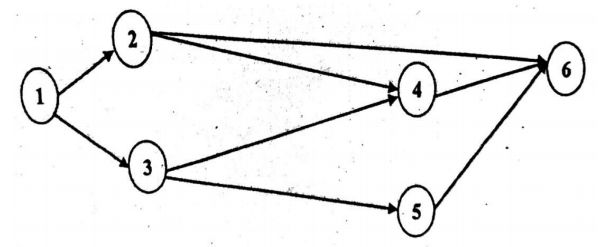Here the time for completion of activities are probabilistic. So, using given values of time we will find the expected time to completion the activities and variance.

$\text {Expected time } \quad t_e = {t_o + 4t_m + t_p \over 6}$ $\text {Variance } V = \Big({t_p - t_o \over 6}\Big)^2$

For each given activity we will calculate the expected time as follows:

Activity t o t m t p $t_e = {t_o + 4t_m + t_p \over 6}$ Variance (V)
1-2 6 9 12 ${6+4 \times 9+12 \over 6} = 9$ $({12-6 \over 6})^2 = 1.000$
1-3 3 4 11 5 1.778
2-4 2 5 14 6 4.000
3-4 4 6 8 6 0.444
3-5 1 1.5 5 2 0.444
2-6 5 6 7 6 0.111
4-6 7 8 15 9 1.778
5-6 1 2 3 2 0.111

Now based on estimate time, we calculate the EST, EFT, LST and LFT for each activity to find out critical path of project as shown below. (Click here to know about calculation of EST, EFT, LST and LFT from CPM numerical)

Activity Duration EST EFT LST LFT Total Float
1-2 9 0 9 0 9 0
1-3 5 0 5 4 9 4
2-4 6 9 15 9 15 0
3-4 6 5 11 9 15 4
3-5 2 5 7 20 22 15
2-6 6 9 15 18 24 9
4-6 9 15 24 15 24 0
5-6 2 7 9 22 24 15

Here the critical path is along the activities 1-2, 2-4, 4-6. So the critical path is 1-2-4-6. Following diagram is prepared to show critical path along with EST and LFT.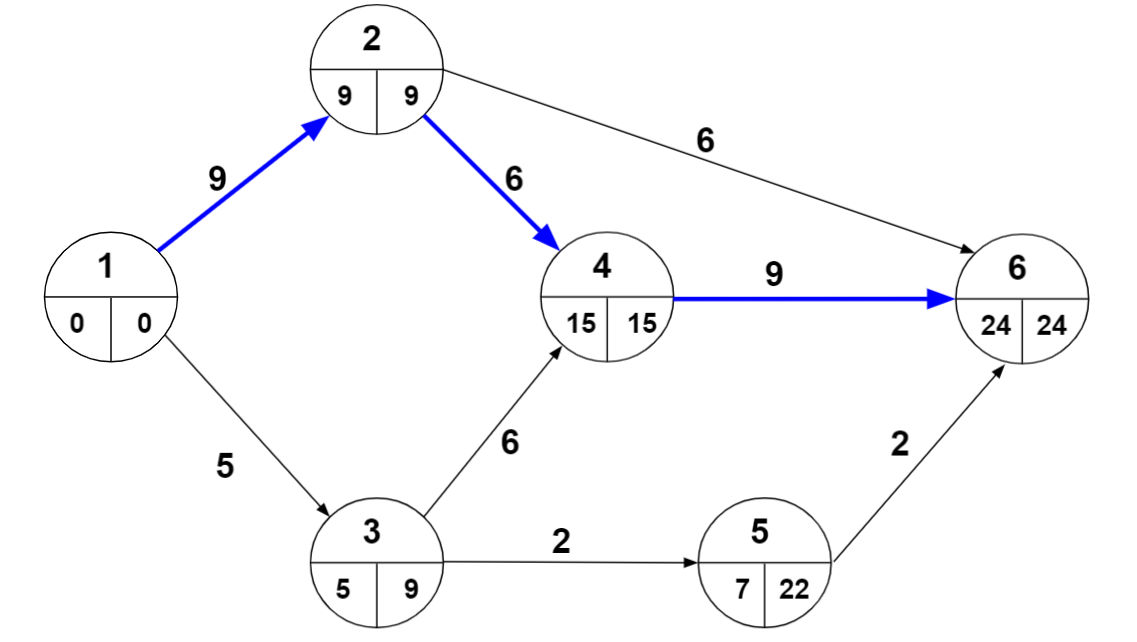$\therefore$ The critical path = 1-2-4-6 with time duration $(t_{cp})$ of 24 days.

Here standard deviation is calculated for activities of critical path. So we get

\begin{aligned} \sigma&= \sqrt{V_1 + V_2 + V_3} \\ \sigma&= \sqrt{1 + 4 + 1.778} \\ &= 2.6034 \end{aligned}

Now the probability of completion of project in that given time (t) of 26 days, can be calculate by below formula,

$Z = {t - t_{cp} \over \sigma} = {26 - 24 \over 2.6034} = 0.7682$

Using table in Appendix-B, we get probability $= 77.8 \%$

As you can see below in Appendix-B the first column Z that is the probability we find out in the example through formula. The second column $\psi(z)$ represent the probability in percentage $(\%)$.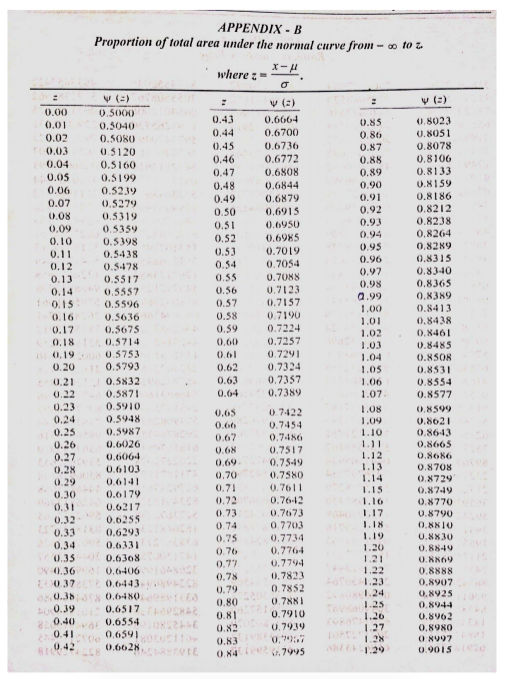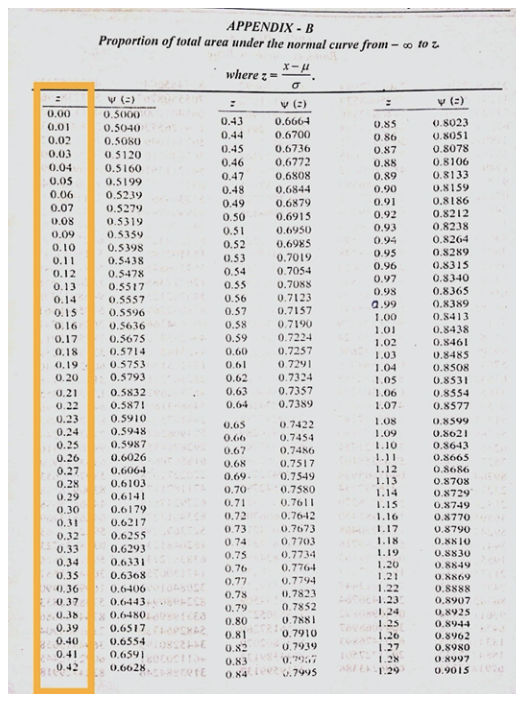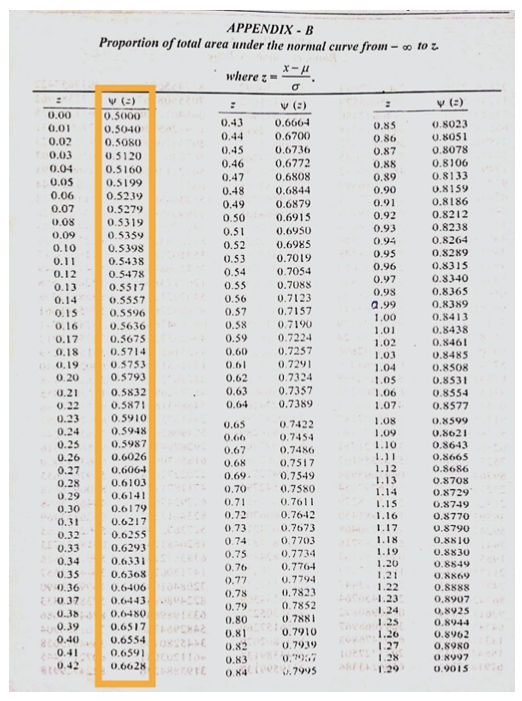As we have value of $Z=0.7682$ now you can see in table we have $Z=0.76$ which has $\psi(z)=0.7764$ and $Z=0.77$ has $\psi(z)=0.7794$ we take average of both we get $\psi(z)=0.7779=77.8\%$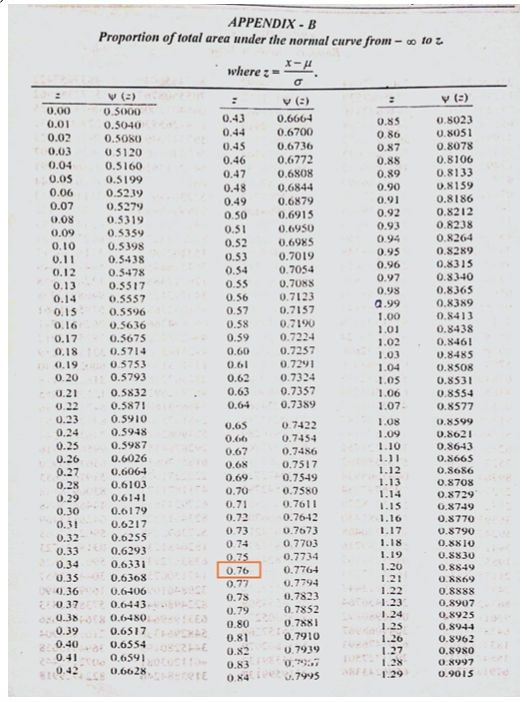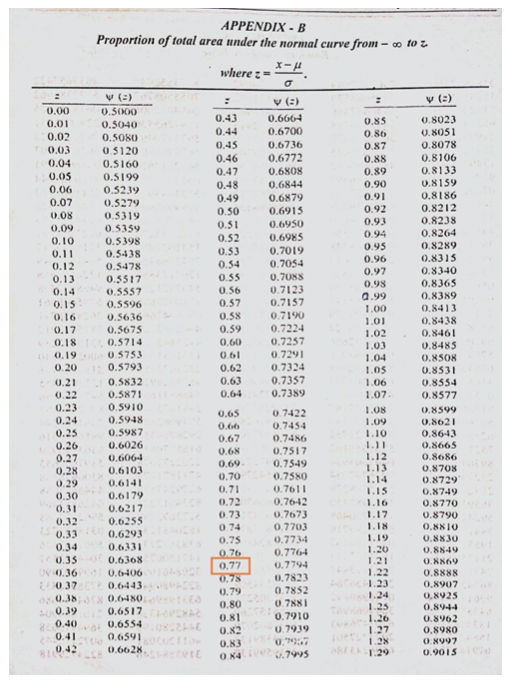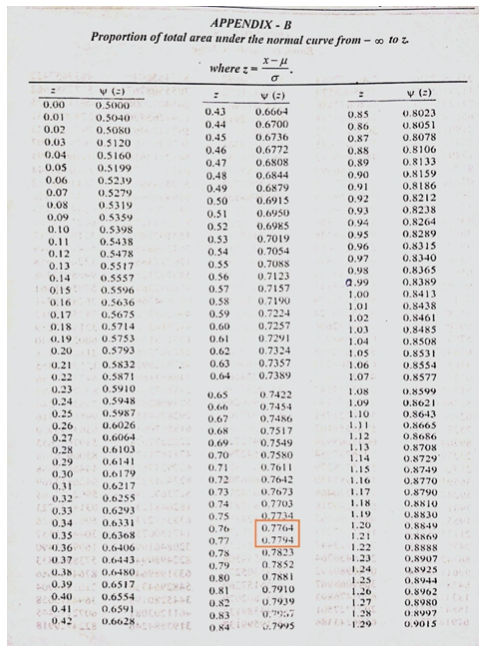As you can see for $Z=0 \space \space$ $\psi(z)=0.5000 \space \space$ which is $50\%$ and for $Z=1 \space \space$ $\psi(z)=0.8413 \space \space$ which is $84.13\%$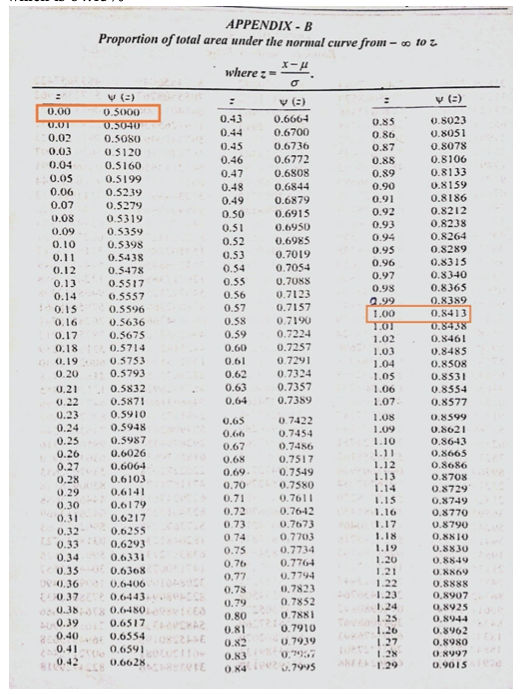## Numerical - North West Corner Method

The North West corner method is one of the methods to obtain a basic feasible solution of the transportation problems (special case of LPP).

We will now see how to apply this very simple method to a transportation problem. We will study steps of this method while applying it in the problem itself.

### Numerical

#### A mobile phone manufacturing company has three branches located in three different regions, say Jaipur, Udaipur and Mumbai. The company has to transport mobile phones to three destinations, say Kanpur, Pune and Delhi. The availability from Jaipur, Udaipur and Mumbai is 40, 60 and 70 units respectively. The demand at Kanpur, Pune and Delhi are 70, 40 and 60 respectively. The transportation cost is shown in the matrix below (in Rs). Use the North-West corner method to find a basic feasible solution (BFS).

Note that all the explanation is provided in “CYAN” colour. You have to write in examination the only thing which are given in this regular colour under each steps(if any), else you can directly solve matrix of the problem as explained here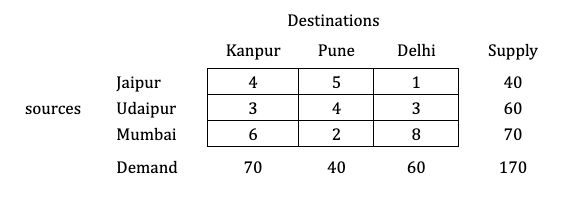Solution:

#### Step 1: Balance the problem

Balance the problem meaning we need to check that if;

$\color{#32c5d4} \Sigma \text { Supply} = \Sigma \text { Demand}$

If this holds true, then we will consider the given problem as a balanced problem.
Now, what if it’s not balanced?

$\color{#32c5d4} \text {i.e., } \Sigma \text { Supply} \not = \Sigma \text { Demand}$

If such a condition occurs, then we have to add a dummy source or market; whichever makes the problem balanced. You can watch a video on this type of numerical, which is known as Unbalanced Transportation Problems.

$\to$ The given transportation problem is balanced.

#### Step 2: Start allocating from North-West corner cell

We will start the allocation from the left hand top most corner (north-west) cell in the matrix and make allocation based on availability and demand.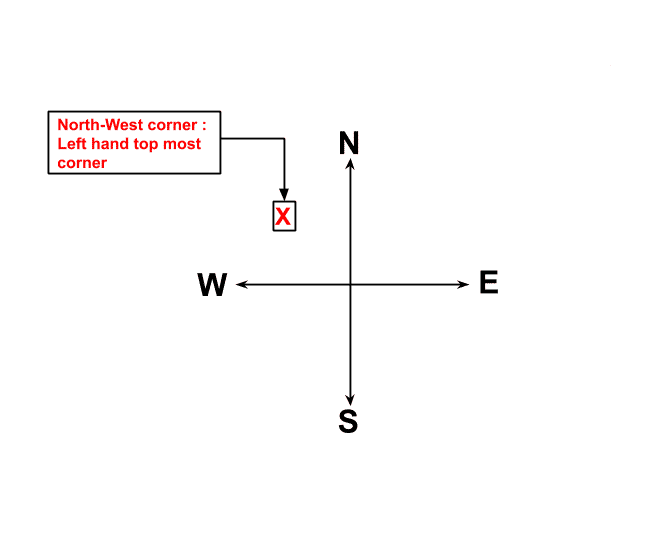Now, verify the smallest among the availability (Supply) and requirement (Demand), corresponding to this cell. The smallest value will be allocated to this cell and check out the difference in supply and demand, representing that supply and demand are fulfilled, as shown below.#### Step 3: Remove the row or column whose supply or demand is fulfilled and prepare a new matrix

As we have fulfilled the availability or requirement for that row or column respectively, remove that row or column and prepare a new matrix, as shown below.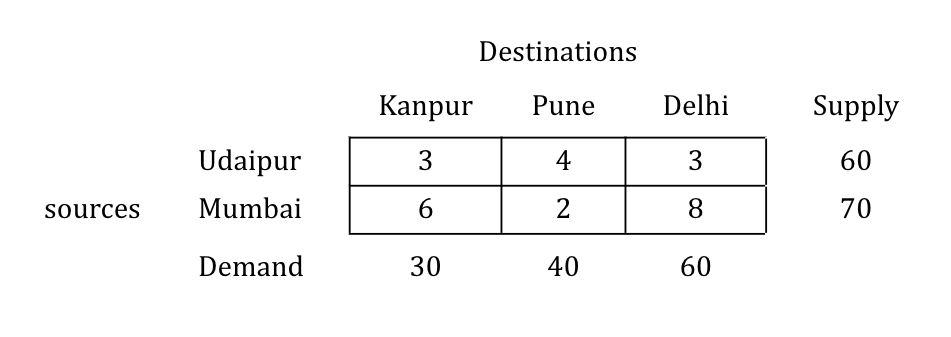#### Step 4: Repeat the procedure until all the allocations are over

Repeat the same procedure of allocation of the new North-west corner so generated and check based on the smallest value as shown below, until all allocations are over.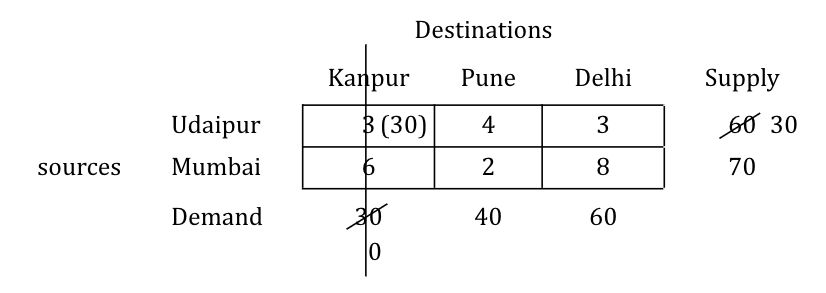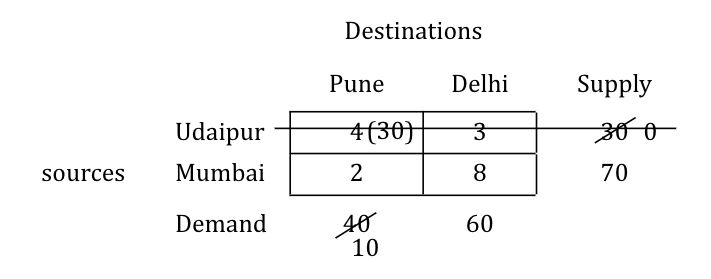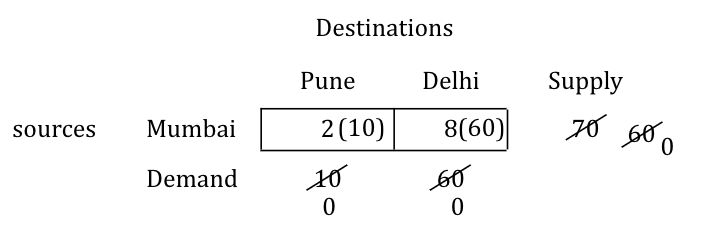#### Step 5: After all the allocations are over, write the allocations and calculate the transportation cost

Once all allocations are over, prepare the table with all allocations marked and calculate the transportation cost as follows.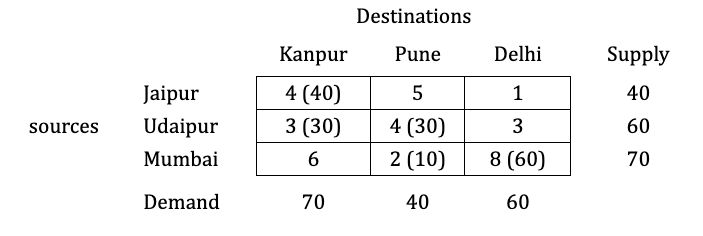\begin{aligned} \to \ \text {Transportation cost} &= (40 \times 40) + (3 \times 30) + (4 \times 30) + (2 \times 10) + (8 \times 60) \\ &= \text {Rs } 870 \end{aligned}

Find solution of same numerical by:

## V-Engine

A V-engine is a two cylinder engine, which has a common crank and the axis of cylinder makes a "V" shape.

Since V-engines have a common crank and the crank revolves in one plane, there is no primary or secondary couple acting on the engine.

Consider a V-engine as shown in fig.1 having common crank OC and two connecting rods CP and CQ. The lines of stroke OP and OQ are inclined to vertical axis OY at an angle ‘α’.Fig-1

Let,

\begin{aligned} m &= \text{mass of reciprocating parts per cylinder, kg}\\ l &= \text{length of connecting rod, m}\\ r &= \text{radius of crank, m}\\ n &= \text{Obliquity ratio }= l/r\\ θ &= \text{Crank angle, measured from vertical axis OY, at any instant}\\ ω &= \text{Angular velocity of crank, rad/s}\\ 2 \alpha &= \text{V-angle i.e. angle between lines of strokes of two cylinders}\\ \end{aligned}

We know that,

• Primary unbalanced force in single cylinder engine is,
$F_P = mr\omega^2 \cos \theta$
• Secondary unbalanced force in single cylinder engine is,
$F_S = mr\omega^2 \bigg({\cos2\theta \over n} \bigg)$

### 1. Primary forces ($F_P$)

#### (i) Primary forces in individual cylinders:

$F_{P1} = mr\omega^2 \cos (\alpha -\theta)\\ F_{P2} = mr\omega^2 \cos (\alpha +\theta)$

#### (ii) Total primary force along Y axis ($F_{PV}$):

As both components $(F_{P1}\sdot \cos\alpha)$ and $(F_{P2} \sdot \cos\alpha)$ are acting in same direction;

\begin{aligned} F_{PV} &= F_{P1} \cos\alpha + F_{P2} \cos\alpha\\ &= mr\omega^2 \cos (\alpha -\theta)\cos\alpha \ - mr\omega^2 \cos (\alpha +\theta)\cos\alpha\\ &= mr\omega^2 \cos\alpha [\cos (\alpha -\theta) + \cos (\alpha+\theta)]\\ &= mr\omega^2 \cos\alpha \sdot (2 \cos\theta \cos\alpha)\\ &= 2mr\omega^2 \cos^2\alpha \cos\theta \end{aligned}

#### (iii) Total primary force along X-axis ($F_{PH}$):

As both components $(F_{P1} \sdot \sin \alpha)$ and $(F_{P2}\sdot \sin \alpha)$ are acting opposite to each other;

\begin{aligned} F_{PH} &= F_{P1} \sin\alpha + F_{P2} \sin\alpha\\ &= mr\omega^2 \cos (\alpha -\theta)\sin\alpha \ - mr\omega^2 \cos (\alpha +\theta)\sin\alpha\\ &= mr\omega^2\sin\alpha [\cos (\alpha -\theta)-\cos (\alpha +\theta)]\\ &= mr\omega^2\sin\alpha \ \sdot (2\sin \theta \sin \alpha)\\ &= 2mr\omega^2\sin^2\alpha\sin \theta \end{aligned}

#### (iv) Resultant primary force ($F_p$):

\begin{aligned} F_p &= \sqrt{(F_{PV})^2 +(F_{PH})^2 }\\ &= \sqrt{(2mr\omega^2 \cos^2\alpha \cos\theta)^2 + (2mr\omega^2\sin^2\alpha\sin \theta)^2}\\ &= 2mr\omega^2\sqrt{(\cos^2\alpha \cos\theta)^2 + (\sin^2\alpha\sin \theta)^2} \end{aligned}

The angle made by resultant primary force with vertical axis is;

\begin{aligned} \beta_P &= \tan^{-1} \bigg ( {F_{PH} \over F_{PV}}\bigg)\\ &= \tan^{-1} \bigg ( {2mr\omega^2\sin^2\alpha\sin \theta \over 2mr\omega^2 \cos^2\alpha \cos\theta} \bigg)\\ &= \tan^{-1} (\tan^2\alpha \ \sdot \ \tan\theta) \end{aligned}

### 2. Secondary forces ($F_S$)

#### (i) Secondary forces in individual cylinder:

$F_{S1} = mr \omega^2 {\cos2(\alpha-\theta) \over n}\\ F_{S2} = mr \omega^2 {\cos2(\alpha+\theta) \over n}\\$

#### (ii) Total secondary force along Y axis ($F_{SV}$):

As both components $(F_{S1}\sdot\cos \alpha)$ and $(F_{S2}\sdot \cos \alpha)$ are acting in same direction;

\begin{aligned} F_{SV} &= F_{S1} \cos \alpha + F_{S2} \cos \alpha\\ &= mr \omega^2 {\cos2(\alpha-\theta) \over n}\cos\alpha + mr \omega^2 {\cos2(\alpha+\theta) \over n}\cos\alpha\\ &= mr \omega^2\cos\alpha \bigg[{\cos2(\alpha-\theta) \over n} + {\cos2(\alpha+\theta) \over n}\bigg]\\ &={1\over n} mr \omega^2\cos\alpha \sdot[2\sdot\cos2\theta\sdot\cos2\alpha]\\ &= {2 \over n}mr \omega^2\cos\alpha\sdot\cos2\alpha\sdot\cos2\theta \end{aligned}

#### (iii) Total secondary force along X-axis ($F_{SH}$):

As both components $(F_{S1}\sdot\sin\alpha)$ and $(F_{S2}\sdot\sin\alpha)$ are acting opposite to each other;

\begin{aligned} F_{SH} &= F_{S1} \sin \alpha - F_{S2} \sin \alpha\\ &= mr \omega^2 {\cos2(\alpha-\theta) \over n}\sin\alpha - mr \omega^2 {\cos2(\alpha+\theta) \over n}\sin\alpha\\ &= mr \omega^2\sin\alpha \bigg[{\cos2(\alpha-\theta) \over n} - {\cos2(\alpha+\theta) \over n}\bigg]\\ &= {1\over n} mr \omega^2\sin\alpha \sdot[2\sdot\sin2\theta\sdot\sin2\alpha]\\ &= {2 \over n}mr \omega^2\sin\alpha\sdot\sin2\alpha\sdot\sin2\theta \end{aligned}

#### (iv) Resultant secondary force ($F_S$):

\begin{aligned} F_s &= \sqrt{(F_{SV})^2 + (F_{SH})^2}\\ &= \sqrt{( {2 \over n}mr \omega^2\cos\alpha\sdot\cos2\alpha\sdot\cos2\theta)^ + ({2 \over n}mr \omega^2\sin\alpha\sdot\sin2\alpha\sdot\sin2\theta)^2}\\ &= {2 \over n} mr\omega^2 \sqrt{(\cos\alpha\sdot\cos2\alpha\sdot\cos2\theta)^2 + (\sin\alpha\sdot\sin2\alpha\sdot\sin2\theta)^2} \end{aligned}

The angle made by resultant secondary force with vertical axis is;

\begin{aligned} \beta_S &= \tan^{-1} \bigg( {F_{SH} \over F_{SV}}\bigg)\\ &= \tan^{-1} \bigg( {{2 \over n}mr \omega^2\sin\alpha\sdot\sin2\alpha\sdot\sin2\theta \over {2 \over n}mr \omega^2\cos\alpha\sdot\cos2\alpha\sdot\cos2\theta} \bigg)\\ &= \tan^{-1}\ (\tan\alpha \sdot \tan2\alpha \sdot \tan2\theta) \end{aligned}

• The design of V-engine is compact compared to multi-cylinder inline engines. So they consume less space.
• Power output of V-engine is more compared to single cylinder engines because the crank receives power from both the cylinders.
• The operation of V-engine is smoother for high speed performance.

## Applications of V-engines

• Because of its compactness, smoother operations and high power output, V-engines are used in sports cars.

## What is the Transportation Model?

Click here to know about Methods to solve Transportation Model Problem provided below here in notes ↓

• Transportation Model is a special case of LPP(Linear Programming Problem) in which the main objective is to transport a product from various sources to various destinations at total minimum cost.

• In Transportation Models, the sources and destinations are known, the supply and demand at each source and destinations are also known.

• It is designed to find the best arrangement for transportation such that the transportation cost is minimum.

## For example:

• Consider three companies (Company1, Company2 and Company3) which produce mobile phones and are located in different regions.

• Similarly, consider three cities (namely CityA, CityB & CityC) where the mobile phones are transported.

• The companies where mobile phones are available are known as sources and the cities where mobile phones are transported are called destinations.

• Let,
Company1 produces a1 units,
Company2 produces a2 units,
Company3 produces a3 units.

• Let,
demand in CityA is b1 units,
demand in CityB is b2 units,
demand in CityC is b3 units.

• The cost of transportation from each source to destination is given in table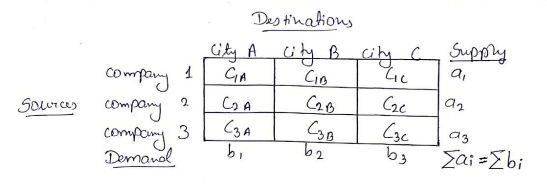• The transportation of mobile phones should be done in such a way that the total transportation cost is minimum.

## Types of transportation problems:

• There are two types of transportation problems:

i) Balanced transportation problem: The sum of supply and sum of demand are same.

$\Sigma \text { Supply} = \Sigma \text { Demand}$

ii) Unbalanced transportation problem: The sum of supply and sum of demand are different.

$\Sigma \text { Supply} \ne \Sigma \text { Demand}$

## Industrial applications of Transportation Model

1. Minimize the transportation cost from source to destination.
2. Determine lowest cost location for new industries, offices, warehouse, etc.
3. Determine the number of products to be manufactured according to demand.
4. Courier Services: Helps in taking proper decisions to find the best route for transportation.

## Basics of Program Evaluation and Review Technique (PERT)

PERT is the technique used to find project completion time of “variable activities”. In PERT, the time is combination of three different time estimations. Following are the three different time estimation:

1. The optimistic time estimate (to): The minimum time required for the completion of the activity as per the predetermined condition.
2. The pessimistic time estimate (tp): The maximum time that activity will take under worst condition.
3. The most likely time estimate (tm): The time an activity will take if executed under normal condition.

## Important terms in PERT analysis:

1. Expected time or average time (te): Since there are three time values available in PERT, average time is to be calculate by following formula:
$t_{e} = {t_o + 4t_m + t_p \over 6}$
1. Variance (V): Variance is given by following formula:
$V = \Big({t_p - t_o \over 6}\Big)^2$
1. Standard Deviation ($\sigma$): Standard deviation is square root of summation of variance of critical activities. It is given by following formula:
$\sigma = \sqrt{V_1 + V_2 + V_3}$
1. Probability of completion of project (z): It is calculated in order to estimate that how many percentages are the chances of completion of project in certain time or given time (t). Let, tcp ​be the time of completion of project on critical path and t is any certain time or given time, then probability of completion of project in that given time t, is given by:
$Z = {t - t_{cp} \over \sigma}$

## Critically Damped System (ξ = 1)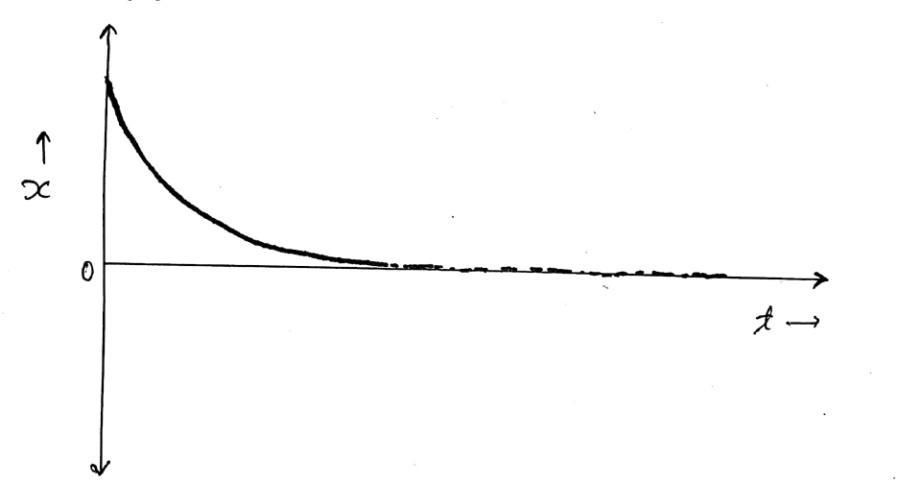Fig.1(Critically Damped System)

• Critically damped system(ξ=1): If the damping factor ξ is equal to one, or the damping coefficient c is equal to critical damping coefficient "cc", then the system is said to be a critically damped system.
$\xi=1 \quad \text OR \quad {c \over c_c} = 1\implies c = c_c$
• Two roots for critically damped system are given by S1 and S2 as below:
$S_1 = \big [-\xi + \sqrt{\xi^2 -1} \big] \omega_n \\ S_2 = \big [-\xi - \sqrt{\xi^2 -1} \big] \omega_n$

• For $ξ=1$; $S_1 = S_2 = -\omega_n$
Here both the roots are real and equal, so the solution to the differential equation can be given by
$x = (A + Bt)e^{-\omega_n t} \quad \quad ...(1)$
• Now differentiating equation (1) with respect to ‘t’, we get:
$\mathring x = Be^{-\omega_nt} - \omega_n(A + Bt)e^{-\omega_nt} \quad \quad ...(2)$
• Now, let at
$t = 0$ : $x = X_0$
$t = 0$ : $\mathring x =0$

• Substituting these values in equation (1):

$X_0 = A \quad ...(3)$
• Same way, from equation (2), we get
\begin{aligned} 0&= B - \omega_n(A + 0) \\ 0&= B - \omega_nA \\ B&= \omega_nA \\ B&= \omega_nX_0 \qquad ...(4) \end{aligned}
• Now putting the values of A and B in equation (1), we get:
\begin{aligned} x&= (X_0 + \omega_nX_0t)e^{-\omega_nt} \\ x&= X_0(1 + \omega_nt)e^{-\omega_nt} \qquad ...(5) \end{aligned}

### Conclusion

• From above equation (5), it is seen that as time t increases, the displacement x decreases exponentially.

• The motion of a critically damped system is aperiodic (aperiodic motion motions are those motions in which the motion does not repeat after a regular interval of time i.e non periodic motion) and so the system does not shows vibrations.

• For critically damped systems, if a system is displaced from its initial position, it will try to reach its mean position in a very short time.

• Critically damped systems are generally seen in hydraulic doors closer as it is necessary for the door to come to its initial position in a very short time.

## Damped free Vibration - Numerical 1

#### A mass of 85 kg is supported on a spring which deflects 18 mm under the weight of the mass. The vibrations of the mass are constrained to be linear and vertical. A dashpot is provided which reduces the amplitude to one-quarter of its initial value in two complete oscillations. Calculate magnitude of the damping force at unit velocity and periodic time of damped vibration.

Solution: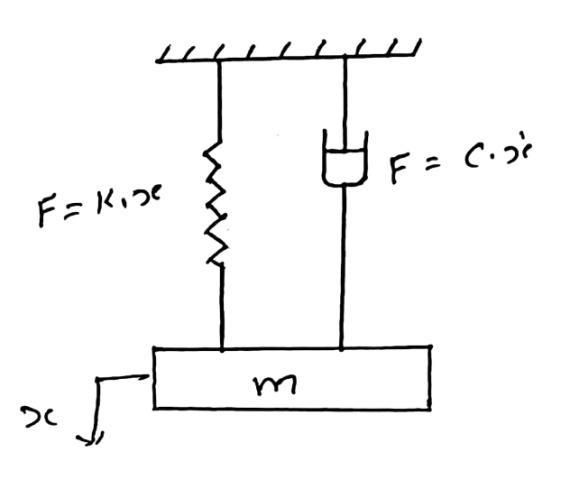Fig-1

Given data:

mass $\ m = 85$ kg
Static deflection, $\ x = 18\ \text{mm} = 0.018\ \text{m}$

Let,
$x_0 =$ initial amplitude
$x_2=$ final amplitude after two complete cycle $= {1\over4}$

Taking ratio,

${x_0 \over x_2}={x_0 \over {1 \over 4} x_0}=4$

#### Damping force:

$\text F = c.\mathring x= c$

Now,

\begin{aligned} \xi= {c \over c_c} \quad \text {OR} \quad &\xi= {c \over 2m\omega_0} \quad (\text {as} \quad c_c=2m\omega_n)\\ \therefore \ &c=(2m\omega_n)\xi \ \dots\dots\dots\dots \ (1) \end{aligned}

#### 1. The Logarithmic decrement:

\begin{aligned} \delta &= {1 \over n} log_e\bigg ({x_0 \over x_n}\bigg)\\ &= {1 \over 2} log_e\bigg ({x_0 \over x_2}\bigg)\\ &= {1 \over 2} log_e(4) \end{aligned}

#### 2. Damping factor:

\begin{aligned} \xi &= {\delta \over \sqrt{4 \pi^2 + {\delta}^2} } \\ &={\delta \over \sqrt{4 \pi^2 + {\delta}^2} }\\ &= 0.1091 \end{aligned}

#### 3. Frequency of undamped free vibration:

The natural circular frequency of vibration is,

\begin{aligned} \omega_n &= {\sqrt{k \over \ m}} \\ &= {\sqrt{m \ g \over \ x \ m}}\\ &= {\sqrt{g \over x}} \\ &= {\sqrt{9.81 \over 0.018}}\\ &= 23.34 \ \text {rad/s} \end{aligned}

From equation (1); we get,
Damping force at unit velocity;

\begin{aligned} \text F = c &= (2m\omega_n)\xi \\ &= (2 \ \text x \ 85\ \text x \ 23.34)\ \text x \ (0.1091) \\ &= 91.67 \ \text N \end{aligned}

The time period of damped vibration is,

\begin{aligned} t_p &= {2\pi \over \omega_d} \\ &= {2\pi \over \omega_n \ \sqrt {1 -\xi^2}} \\ &= {2\pi \over (23.34) \ \sqrt {1 -(0.1091)^2}} \\ &=0.2708 \ \text {sec} \end{aligned}

## Over-Damped System (​ξ>1)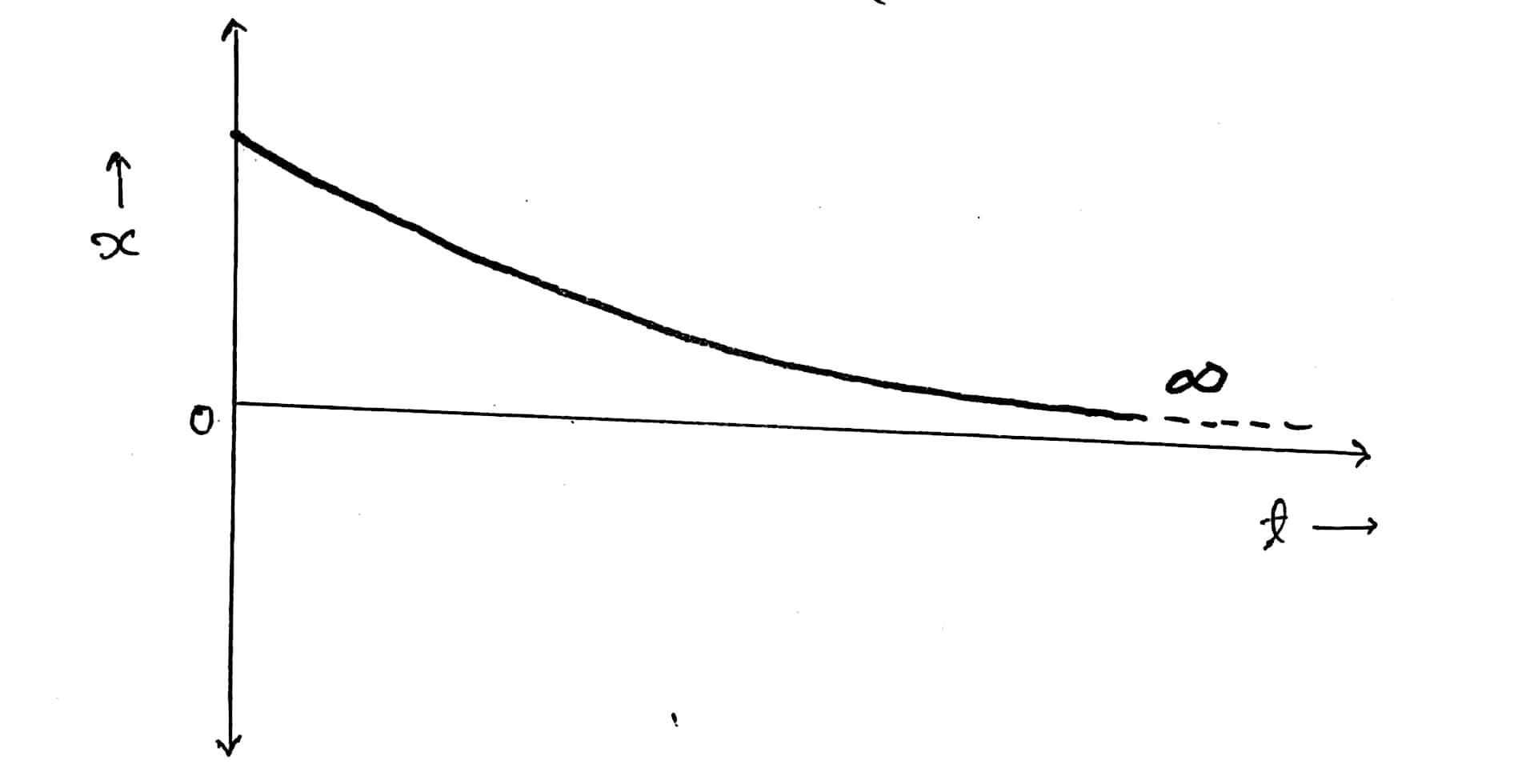Fig-1 (Over damped system)

We know that the characteristic equation of the damped free vibration system is,

$mS^2 + cS + K = 0$

This is a quadratic equation having two roots $S_1$ and $S_2$;

$S_{1,2} = {-c \over 2m} \pm \sqrt{\bigg({-c \over 2m}\bigg)^2 - {K \over m}}$

In order to convert the whole equation in the form of $\xi$ , we will use two parameters, critical damping coefficient '$c_c$' and damping factor '$\xi$'. So the roots $S_1$ and $S_2$ can be written as follows;

\begin{aligned} \xi= {c \over c_c} \quad \text {OR} \quad \xi &= {c \over 2m\omega_0} \quad (\text {as} \quad c_c=2m\omega_n)\\ \therefore {c \over 2m} &= \xi \omega_n \end{aligned}

where, $\omega_n$ = natural frequency of undamped free vibration = $\sqrt{K \over m}$ rad/s

$\therefore \omega_n^2 = {K \over m}$

So we can write roots $S_1$ and $S_2$ and as;

\begin{aligned} S_{1,2} &= -\xi \omega_n \pm \sqrt{(\xi \omega_n)^2 - \omega_n^2}\\ \therefore S_{1,2} &= [-\xi \pm \sqrt{\xi^2 - 1}]\omega_n\\ \therefore \ \ S_{1} &= [-\xi + \sqrt{\xi^2 - 1}]\omega_n\\ \text{And, }\\ S_{2} &= [-\xi - \sqrt{\xi^2 - 1}]\omega_n \end{aligned}

## Overdamped system (ξ>1)

If the damping factor ‘$\xi$’ is greater than one or the damping coefficient ‘$c$’ is greater than critical damping coefficient ‘$c_c$’, then the system is said to be over-damped.

$\xi > 1 \quad \text{Or} \quad {c \over c_c}\quad \text{Or} \quad c > c_c$

In overdamped system, the roots are given by;

\begin{aligned} S_{1} &= [-\xi + \sqrt{\xi^2 - 1}]\omega_n\\ \text{And, }\\ S_{2} &= [-\xi - \sqrt{\xi^2 - 1}]\omega_n \end{aligned}

For $\xi > 1$ , we get $S_1$ and $S_2$ as real and negative so we get,

\begin{aligned} x &= Ae^{S_1t} + Be^{S_2t}\\ \therefore x &= Ae^{[-\xi + \sqrt{\xi^2-1}]\omega_nt} + Be^{[-\xi - \sqrt{\xi^2-1}]\omega_nt} \quad \dots \dots \text{(1)} \end{aligned}

Now differentiating equation (1) with respect to ‘t’, we get;

\begin{aligned} \mathring x = &Ae^{[-\xi + \sqrt{\xi^2-1}]\omega_nt}[-\xi + \sqrt{\xi^2-1}]\omega_n \ + \\ &Be^{[-\xi - \sqrt{\xi^2-1}]\omega_nt} [-\xi - \sqrt{\xi^2-1}]\omega_n\quad \dots \dots \dots \text{(2)} \end{aligned}

Now, let at
$t = 0$ : $x = X_0$
$t = 0$ : $\mathring x =0$

Substituting this value in equation (1) we get;

$X_0 = A+B\quad \dots \dots \dots \text{(3)}$

Substituting this value in equation (2) we get;

$0= A[-\xi + \sqrt{\xi^2-1}]\omega_n + B[-\xi - \sqrt{\xi^2-1}]\omega_n \quad \dots \dots \text{(4)}$

From equation (3), $B= X_0 - A$ and putting value of B in (4);

\begin{alignedat}{2} &\therefore \qquad 0 &=& \ A[-\xi + \sqrt{\xi^2-1}]\omega_n + (X_0 - A)[-\xi - \sqrt{\xi^2-1}]\omega_n\\ &\therefore \qquad 0 &=& \ -A\xi + A\sqrt{\xi^2-1} + X_0 [-\xi - \sqrt{\xi^2-1}]+A\xi+A\sqrt{\xi^2-1}\\ &\therefore \qquad 0 &=& \ 2A\sqrt{\xi^2-1} + X_0 [-\xi - \sqrt{\xi^2-1}]\\ &\therefore 2A\sqrt{\xi^2-1} \ &=& \ X_0 [\ \xi + \sqrt{\xi^2-1}]\\ &\therefore \qquad A &=& \ {X_0 [\xi + \sqrt{\xi^2-1}] \over 2\sqrt{\xi^2-1} }\quad \dots \dots \text{(5)} \end{alignedat}

Now,
Putting $A=X_0-B$, in equation (4);

\begin{alignedat}{2} &\therefore \qquad 0 &=& \ (X_0-B)[-\xi + \sqrt{\xi^2-1}]\omega_n + B[-\xi - \sqrt{\xi^2-1}]\omega_n\\ &\therefore \qquad 0 &=& \ X_0[-\xi + \sqrt{\xi^2-1}] + B\xi - B\sqrt{\xi^2-1} - B\xi - B\sqrt{\xi^2-1}\\ &\therefore \qquad 0 &=& \ X_0[-\xi + \sqrt{\xi^2-1}] - 2B\sqrt{\xi^2-1}\\ &\therefore 2B\sqrt{\xi^2-1} &=& \ X_0[-\xi + \sqrt{\xi^2-1}]\\ &\therefore \qquad B &=& \ {X_0[-\xi + \sqrt{\xi^2-1}] \over 2\sqrt{\xi^2-1}} \quad \dots \dots \text{(6)} \end{alignedat}

Now putting equation (5) and (6) in equation (1), we get;

\begin{aligned} x = &{X_0 [\xi + \sqrt{\xi^2-1}] \over 2\sqrt{\xi^2-1} } e^{[-\xi + \sqrt{\xi^2-1}]\omega_nt} +\\ &{X_0[-\xi + \sqrt{\xi^2-1}] \over 2\sqrt{\xi^2-1}} e^{[-\xi - \sqrt{\xi^2-1}]\omega_nt}\\ x = &{X_0 \over 2\sqrt{\xi^2-1}} \bigg [\bigg (\xi + \sqrt{\xi^2-1} \bigg) e^{[-\xi + \sqrt{\xi^2-1}]\omega_nt} + \\ &\bigg (-\xi + \sqrt{\xi^2-1} \bigg ) e^{[-\xi - \sqrt{\xi^2-1}]\omega_nt} \bigg ] \end{aligned}

Above equation represents the equation of motion for overdamped system.

The motion obtained by above equation is aperiodic (aperiodic motion motions are those motions in which the motion does not repeats after a regular interval of time i.e non periodic motion) and so the system does not shows vibrations.

This type of system does not show much damping, so this systems are used very rarely.

## Static and Dynamic Balancing

When machine is in working condition different forces are acting on it which may cause machine to vibrate and cause damage to machine parts. The different forces acting on machine parts are static forces and dynamic forces.

### Static force

The force which depends on weight of a body, is known as static force. (Generally, a static force acts when vibrations occurs in same plane.)

### Dynamic or Inertia force

The force which depends on acceleration of a body, is known as dynamic force. (Generally, dynamic force acts when vibrations occurs in different planes.)

Due to these forces, the efficiency of the system decreases and life span of the system also decreases.
Due to these forces, the machine starts vibrating and sometimes when the vibrations increases, the machine would lift from it’s position and cause damage to other machine or human. So to avoid this, foundation is made below the machine (as you can see in the Fig-1 below), which absorbs the vibration and protects the machine against causing damage. Thus, balancing of the machine is required.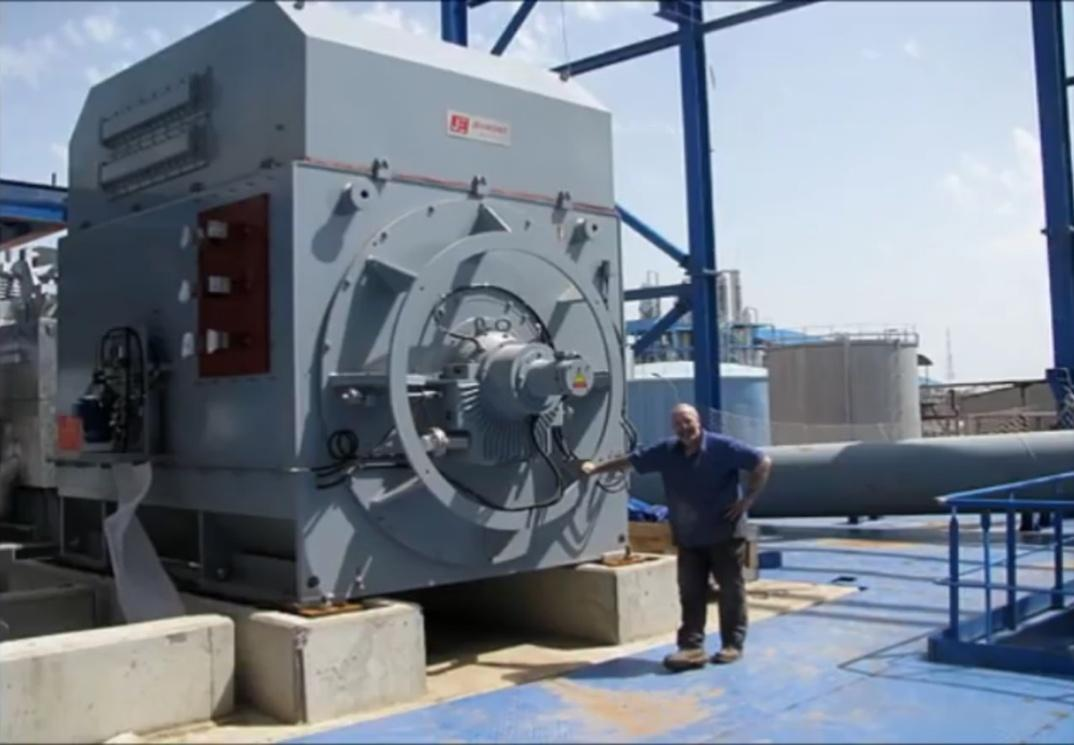Fig-1

### Balancing

Balancing is the process of eliminating, the effect of static forces and dynamic forces acting on machine components.

## When is system said to be unbalanced?

In any system with one or more rotating masses, if the centre of mass of the system does not lie on the axis of rotation, then the system is said to be unbalanced.

As you can see in the Fig-2 we have a rotor which is mounted on a shaft and the shaft has its own axis of rotation. Now you can see that the C.G(centre of gravity) of the rotor is at a distance r from the axis of rotation of the shaft, so when the rotor will start to rotate, a centrifugal force will act on it which is in the outward direction as you can see in Fig-3. Due to this force our system will become unbalanced and it will start to vibrate.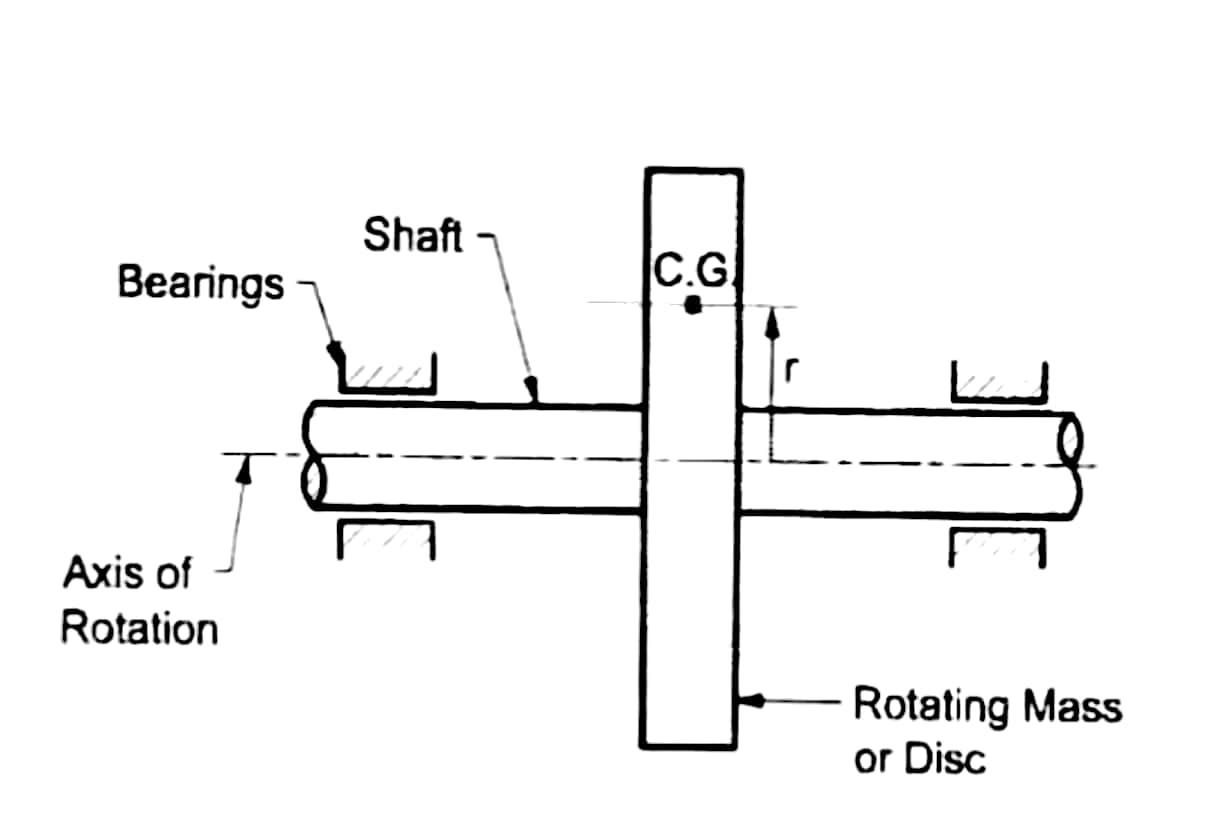Fig-2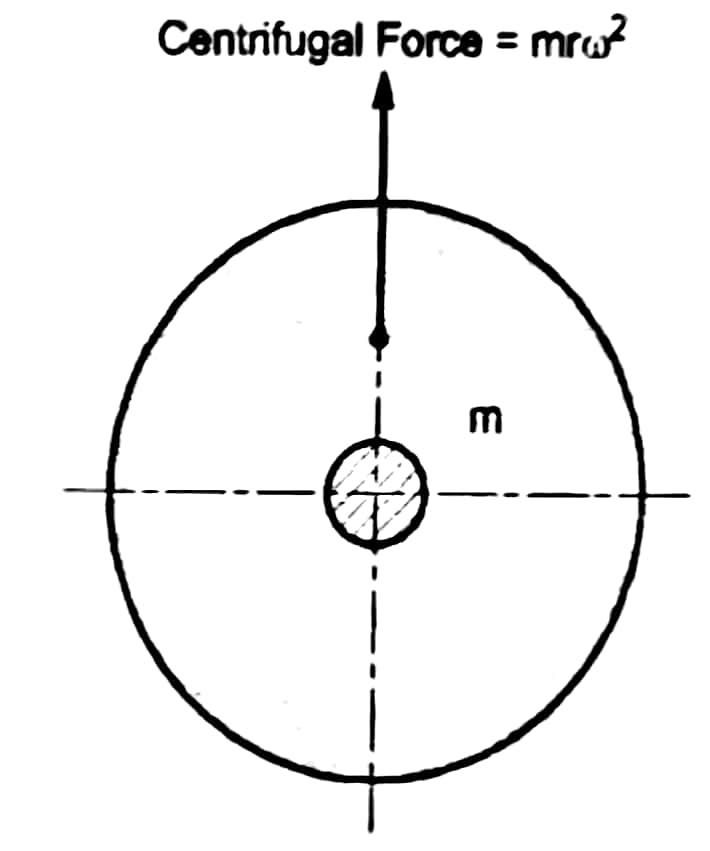Fig-3

### Static balancing

A system is said to be statically balanced, if the centre of masses(C.G) of the system lies on the axis of rotation.

#### Condition for Static Balancing

The resultant of all the centrifugal forces (dynamic forces) acting on the system during rotation must be zero.

\begin{aligned} \small ∑ \text {Centrifugal forces acting on the system} &= \text {zero}\\ i.e. \ +mrω^2 – mrω^2 &= 0 \end{aligned}

### Dynamic balancing

A system is said to be dynamically balanced, if it satisfies following two conditions:-

1. The resultant of all the dynamic forces acting on the system during rotation must be zero.
$∑ \text {Dynamic forces acting on the system} = \text {zero}$
1. The resultant couple due to all the dynamic forces acting on the system during rotation, about any plane, must be zero.
$∑ \text {couple} = \text {zero}$

## Logarithmic Decrement (​δ)
fig-1: displacement V/s time curve for under damped system

### Logarithmic decrement

Logarithmic decrement is defined as the natural logarithm of the ratio of successive amplitude on the same side of mean position.

The rate of decay in the amplitudes of under-damped system is measured by the parameter known as logarithmic decrement.

Rate of decay in amplitudes depends on the amount of damping present in the system. So if the damping is more, then the rate of decay will also be more.

Let A and B are the two points on the successive cycles which shows maximum deflection as shown in figure.

The periodic time:

\begin{aligned} t_p &= t_2 − t_1 \\ &= {2 \pi \over \omega_d} \\ &= {2 \pi \over \big(\sqrt {1-\xi^2}\big) \ \omega_n} \end{aligned}

The amplitude at time $t_1$ and $t_2$ are:

$x_1 = Xe^{-\xi \omega_n t_1} [sin(\omega_d t_1 + \varnothing)]$

And,

\begin{aligned} x_2 &= Xe^{-\xi \omega_n t_2} [sin(\omega_d t_2 + \varnothing)] \\ \therefore \quad x_2 &= Xe^{-\xi \omega_n (t_1 + t_p)} [sin \{ \omega_d (t_1 + t_p) + \varnothing \}] \\ \therefore \quad x_2 &= Xe^{-\xi \omega_n (t_1 + t_p)} [sin ( \omega_d t_1 + \omega_d t_p + \varnothing)] \\ \therefore \quad x_2 &= Xe^{-\xi \omega_n (t_1 + t_p)} [sin ( \omega_d t_1 + \omega_d \bigg({2 \pi \over \omega_d}\bigg) + \varnothing)] \\ \therefore \quad x_2 &= Xe^{-\xi \omega_n (t_1 + t_p)} [sin ( \omega_d t_1 + 2 \pi + \varnothing)] \\ \therefore \quad x_2 &= Xe^{-\xi \omega_n (t_1 + t_p)} [sin \{ 2 \pi + (\omega_d t_1 + \varnothing) \}] \\ \therefore \quad x_2 &= Xe^{-\xi \omega_n (t_1 + t_p)} [sin (\omega_d t_1 + \varnothing)] \\ \end{aligned}

Now,
Taking ratio, we get;

\begin{aligned} {x_1 \over x_2} &= {Xe^{-\xi \omega_n t_1} [sin(\omega_d t_1 + \varnothing)] \over Xe^{-\xi \omega_n (t_1 + t_p)} [sin (\omega_d t_1 + \varnothing)] }\\ \therefore \quad \quad {x_1 \over x_2} &= e^{-\xi \omega_n (t_1-t_1-t_p)} \\ \therefore \quad \quad {x_1 \over x_2} &= e^{\xi \omega_n t_p} \end{aligned}

Now,
The logarithmic decrement is given by;

\begin{aligned} \delta &= log_e \bigg({x_1 \over x_2}\bigg) \\ \therefore \quad \delta &= log_e (e^{\xi \omega_n t_p}) \\ \therefore \quad \delta &= \xi \omega_n t_p \\ \therefore \quad \delta &= \xi \omega_n {2 \pi \over \big(\sqrt {1-\xi^2}\big) \ \omega_n} \\ \therefore \quad \delta &= {2 \pi \xi\over \big(\sqrt {1-\xi^2}\big)} \end{aligned}

The logarithmic decrement can also be determined as follows;

\begin{aligned} \delta &= log_e \bigg({x_0 \over x_1}\bigg)= log_e \bigg({x_1 \over x_2}\bigg)= log_e \bigg({x_2 \over x_3}\bigg) = \dotso = log_e \bigg({x_{n-1} \over x_n}\bigg) \\ \text {Adding upto 'n' terms}\\ n\delta &= log_e \bigg({x_0 \over x_1} \bigg) + log_e \bigg({x_1 \over x_2}\bigg) + log_e \bigg({x_2 \over x_3}\bigg) + \dotso + log_e \bigg({x_{n-1} \over x_n}\bigg) \\ \therefore \ \ n\delta &= log_e \bigg({x_0 \over x_1} \ . {x_1 \over x_2} \ . {x_2 \over x_3} \ . \dots \ . {x_{n-1} \over x_n}\bigg) \\ \text {Or} \qquad \\ n\delta &= log_e \bigg({x_0 \over x_n}\bigg) \\ \therefore \quad \delta &= {1 \over n} log_e \bigg({x_0 \over x_n}\bigg) \end{aligned}

where,
$x_0$ = amplitude at the starting position
$x_n$ = amplitude after ‘n’ cycles

## Under-Damped System (​ξ < 1)
Fig-1

## Underdamped system $\ (\xi < 1)$

If the damping factor $\ \xi$ is less than one or the damping coefficient $c$ is less than critical damping coefficient $c_c$, then the system is said to be an under-damped system.

$\xi < 1 \quad \text OR \quad {c \over c_c} < 1\implies c < c_c$
• We know that roots of differential equations are:
$S_1 = \big [-\xi + \sqrt{\xi^2 -1} \big] \omega_n \\ S_2 = \big [-\xi - \sqrt{\xi^2 -1} \big] \omega_n$

• But for $\ \xi < 1$; the roots for under-damped system are given by $S_1$ and $S_2$ as below:
\begin{aligned} S_1 = & \big [-\xi + i \sqrt{(1 - \xi^2)}] \omega_n \\ S_2 = & \big [-\xi - i \sqrt{(1 - \xi^2)}] \omega_n \\ \end{aligned}

Where $i = \sqrt{-1}$ is the imaginary unit of complex root

• The roots are complex and negative, so the solution of differential equation is given by
$\begin{array}{rl}x& =A{e}^{{S}_{1}t}+B{e}^{{S}_{2}t}\\ \therefore x& =A{e}^{\left[-\xi +i\sqrt{\left(1-{\xi }^{2}\right)}\right]{\omega }_{n}t}+B{e}^{\left[-\xi -i\sqrt{\left(1-{\xi }^{2}\right)}\right]{\omega }_{n}t}\\ \therefore x& =A\mathrm{.}{e}^{-\xi {\omega }_{n}t}\mathrm{.}{e}^{i\sqrt{\left(1-{\xi }^{2}\right)}{\omega }_{n}t}+B\mathrm{.}{e}^{-\xi {\omega }_{n}t}\mathrm{.}{e}^{-i\sqrt{\left(1-{\xi }^{2}\right)}{\omega }_{n}t}\\ \end{array}$